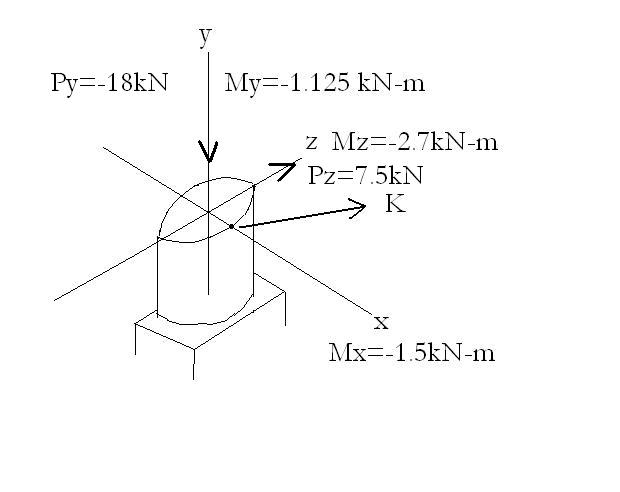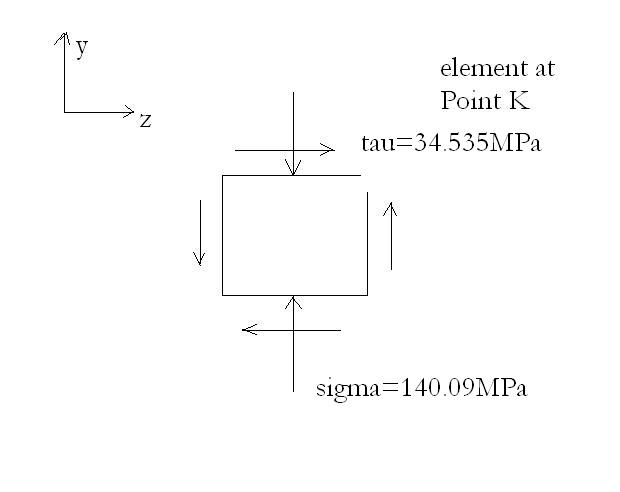# Principle stresses and maximum shearing stress in a cylindrical shaft. Pl help!

## Homework Statement

Nevermind the rough picture, the shaft is cylindrical. Knowing that the post has a diameter of 60mm, determine the principle stresses and the maximum shearing stress at point K.## Homework Equations

These are equations for your reference:

$\sigma = My/I$
$\tau = Tc/J$
Where M is bending moment, T is twisting torque. J is polar moment of inertia. c is radius of cylindrical shaft. y is distance of point from neutral axis.

## The Attempt at a Solution

I find:

J = pi/2 . c^4 = 1.27e-6 m^4; I = J/2 = 6.36e-7 m^4

$\tau_K = Tc/J = M_yc/J = 1.125e3\cdot0.03/ 1.27e-6 = 26.56MPa$

$(\sigma_K)_y= M_zc/I = -2.7e3\cdot0.03/ 6.36e-7 = -127.32 MPa$

$(\sigma_K)_z = 0$ right?!?!

Because of the shearing force, $P_z, \tau = 3/2 \cdot P/A = 3/2 \cdot 7.5e3/(\pi /2 \times .03^2) = 7.965 MPa$
So, $\tau_{total}= 26.57MPa + 7.965MPa = 34.535MPa$
Now, because of $M_x$ there won't be any normal longitudinal stress at K because K lies on neutral axis, right? So the only normal longitudinal stress is due to $M_z$.

Now, because of P_y there will be compressive stress = $P_y/A = -18e3/(\pi / 2 \times .03^2) = -12.73MPa$
This I add with the normal longitudinal stress $\sigma_y=-127.36MPa$ calculated earlier.
So, $(\sigma_y)_{total} = -127.36 - 12.73MPa = - 140.09MPa$

So this is the diagram for the stress distribution on an element at point K:So the principle stresses are found to be:

$\tau_{max} = \sqrt{((\sigma_y-\sigma_z)/2)^2 + (\tau_{yz})^2} = \sqrt{((-140.09-0)/2)^2 + 34.535^2} = 78.09 MPa.$

$And, \sigma_{max,min} = \sigma_{ave} \pm \tau_{max} = (-140.09/2 \pm 78.09) MPa = -148.135MPa, 8.045MPa$

The answers in the back of the text are : 6.45MPa, -140.0MPa for 73.3MPa. I am not sure which value is for which stress. I guess the first and last values are for the principle stresses and the middle value is for the maximum shearing stress.

Last edited: